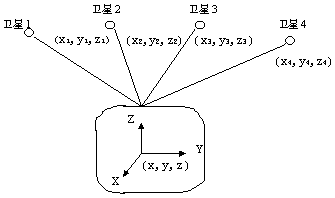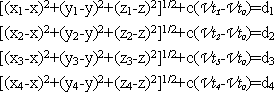# Introduction to the basic principle of GPS navigation system and GPS positioning principle

GPS is a radio navigation system consisting of 27 navigation satellites launched by the US Department of Defense. It consists of satellite, ground station and receiver. On the ground, as long as a GPS receiver is used to measure the time when 3-4 satellite signals arrive at the receiver, the latitude, longitude, altitude, and time of the receiver's location (which can be anywhere on the ground or in the sky) can be accurately estimated. , speed of movement and other data.

1, the composition of the GPS system

GPS consists of three separate parts:

â— Space: 21 working satellites and 3 spare satellites.

â— Ground support system: 1 main control station, 3 injection stations, 5 monitoring stations.

â— User equipment part: Receive GPS satellite transmission signals to obtain necessary navigation and positioning information, and complete navigation and positioning work through data processing.

The GPS receiver hardware is generally composed of a host, an antenna, and a power source.

2, GPS positioning principle

The basic principle of GPS positioning is based on the instantaneous position of the satellite moving at high speed as the known starting data, and the method of spatial distance resection is used to determine the position of the point to be measured. As shown in the figure, it is assumed that the GPS receiver is placed on the ground to be measured at time t, and the time Î”t at which the GPS signal arrives at the receiver can be determined. Together with other data such as the satellite ephemeris received by the receiver, the following four can be determined. Equations:The coordinates x, y, z and Vto of the points to be measured in the above four equations are unknown parameters, where di = c Î”ti (i = 1, 2, 3, 4).

Di (i = 1, 2, 3, 4) is the distance between satellite 1, satellite 2, satellite 3, satellite 4 and the receiver, respectively.

Î”ti (i=1, 2, 3, 4) are the times that the signals of satellite 1, satellite 2, satellite 3, and satellite 4 arrive at the receiver, respectively.

c is the propagation speed of the GPS signal (ie, the speed of light).

The meaning of each parameter in the four equations is as follows:

X, y, and z are spatial rectangular coordinates of the coordinates of the point to be measured.

Xi, yi, zi (i = 1, 2, 3, 4) are space rectangular coordinates of satellite 1, satellite 2, satellite 3, and satellite 4 at time t,

It can be obtained from satellite navigation messages.

Vt i (i = 1, 2, 3, 4) is the clock difference of the satellite clocks of satellite 1, satellite 2, satellite 3, and satellite 4, respectively, provided by the satellite ephemeris.

Vto is the clock difference of the receiver.

From the above four equations, the coordinates x, y, z of the point to be measured and the clock difference Vto of the receiver can be solved.

3. The basic principle of GPS navigation system

It can be seen that the role of the satellite part of the GPS navigation system is to continuously transmit navigation messages. However, since the clock used by the user's receiver is not always synchronized with the satellite onboard clock, in addition to the user's three-dimensional coordinates x, y, z, a Î”t, that is, the time difference between the satellite and the receiver is introduced as an unknown number. Then use four equations to solve the four unknowns. So if you want to know where the receiver is, you must be able to receive at least 4 satellite signals.

The GPS receiver can receive time information accurate to the nanosecond level that can be used for timing; a forecast ephemeris used to predict the approximate location of the satellite in the next few months; and a broadcast ephemeris used to calculate the satellite coordinates required for positioning. The accuracy is from a few meters to tens of meters (different satellites, changing at any time); and GPS system information, such as satellite status.

The GPS receiver measures the distance from the satellite to the receiver. It is called pseudorange because it contains the error of the receiver satellite clock and the atmospheric propagation error. The pseudorange measured by the 0A code is called the UA code pseudorange, and the precision is about 20 meters. The pseudorange measured by the P code is called the P code pseudorange, and the precision is about 2 meters.

The GPS receiver decodes the received satellite signal or uses other techniques to remove the information modulated on the carrier and recover the carrier. Strictly speaking, the carrier phase should be referred to as the carrier beat frequency phase, which is the difference between the received satellite signal carrier phase affected by the Doppler shift and the phase of the receiver local oscillator generated signal. Generally, the epoch time determined by the receiver clock is measured, and the tracking of the satellite signal is kept, and the phase change value can be recorded, but the initial phase values â€‹â€‹of the receiver and the satellite oscillator at the start of observation are unknown. The phase integer of the epoch is also unknown, that is, the ambiguity of the whole week can only be solved as a parameter in data processing. The accuracy of the phase observation is as high as millimeter, but the premise is that the whole-circumference ambiguity is solved. Therefore, the phase observation value can be used only when the relative positioning is performed and there is a continuous observation value, and the positioning accuracy is better than the meter level. Phase observations can be used.

According to the positioning method, GPS positioning is divided into single point positioning and relative positioning (differential positioning). Single point positioning is a way to determine the position of the receiver based on the observation data of a receiver. It can only be measured by pseudo-range observation and can be used for rough navigation and positioning of vehicles and ships. Relative positioning (differential positioning) is a method of determining the relative position between observation points based on observation data of two or more receivers. It can use both pseudo-range observation and phase observation. Geodesy or engineering measurement should be performed. Phase observations are used for relative positioning.

The GPS observations include errors such as the clock difference of the satellite and the receiver, the atmospheric propagation delay, and the multipath effect. They are also affected by the satellite broadcast ephemeris error during the positioning calculation. Most of the common errors are caused by relative positioning. Offset or weaken, so the positioning accuracy will be greatly improved. The dual-frequency receiver can offset the main part of the ionospheric error in the atmosphere according to the observation of two frequencies. When the accuracy is high and the distance between receivers is far away (the atmosphere is obviously different) ), should use dual-frequency receiver.

Ni-Fe Battery 250~400ah

Solar Power Battery,Nife Batteries For Solar,Nickel Iron Battery 400Ah,Ni-Fe Battery 250~400Ah

Henan Xintaihang Power Source Co.,Ltd , https://www.taihangbattery.com